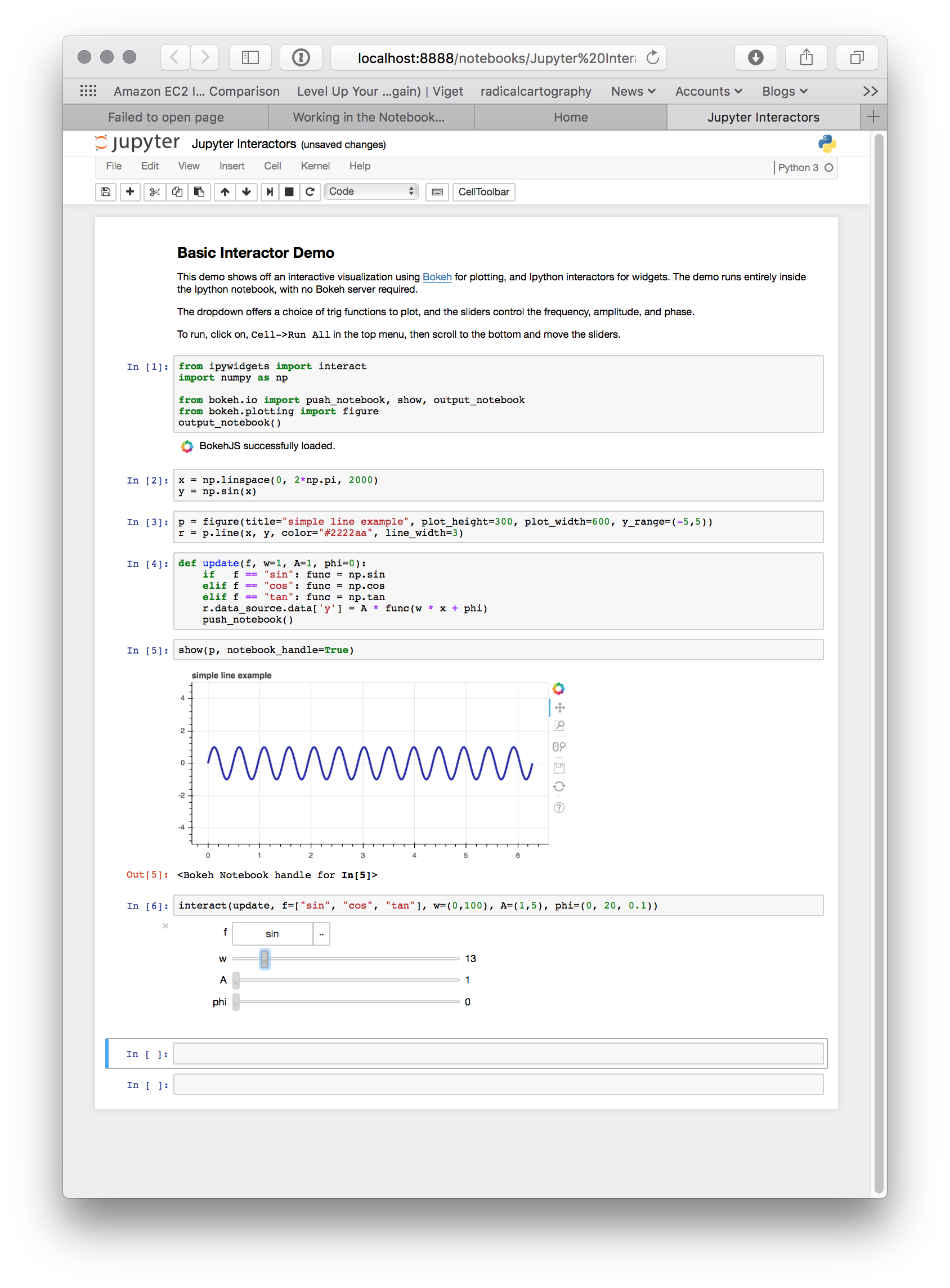Working in the Notebook¶

Inline Plots¶

To display Bokeh plots inline in an Jupyter notebook, use the output_notebook() function from bokeh.io instead of (or in addition to) the output_file() function we have seen previously. No other modifications are required. When show() is called, the plot will be displayed inline in the next notebook output cell. You can see a screenshot below: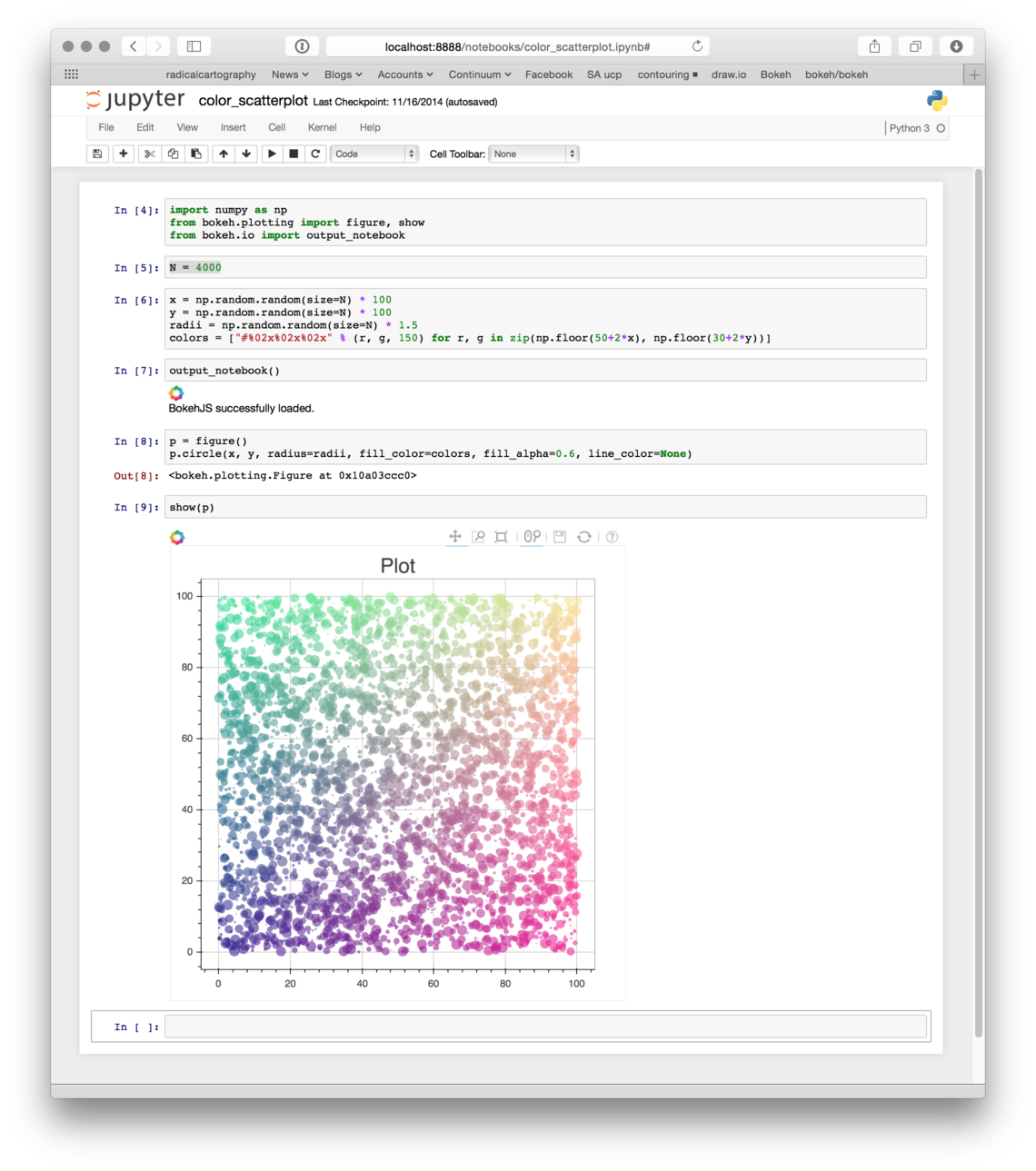Note

As a convenience, output_notebook() is also importable from the bokeh.charts and bokeh.plotting modules.

Notebook Handles¶

It is possible to update a previously shown plot in-place. When the argument notebook_handle=True is passed to show() then a handle object is returned. This handle object can be used with the push_notebook() function to update the plot with any recent changes to plots properties, data source values, etc.

The following screenshots walk through the basic usage of notebook handles.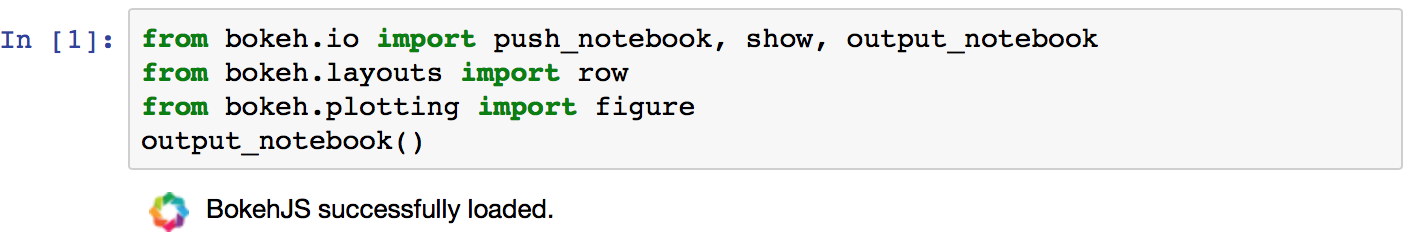• Next, create some plots, and make sure to pass handle_notebook=True to show():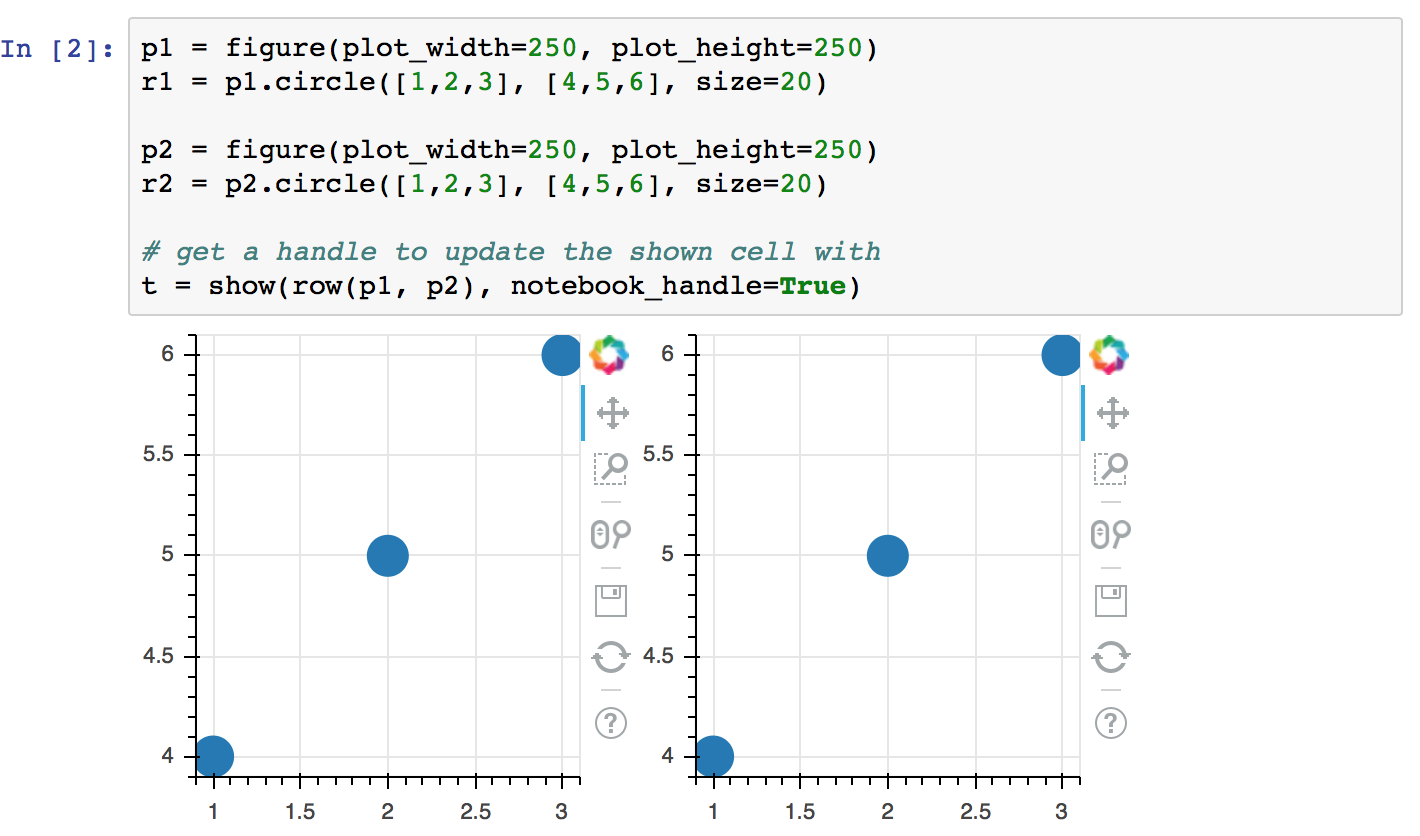• Looking at the handle, see that it is associated with the output cell for In that was just displayed: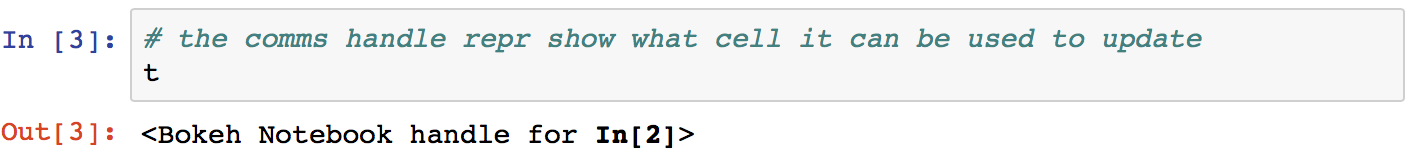• Now, update any properties of the plot, then call push_notebook() with the handle: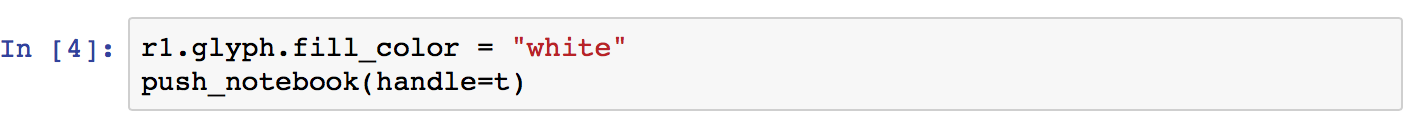• After doing so, note that the earlier output cell for In has changed (without being re-executed)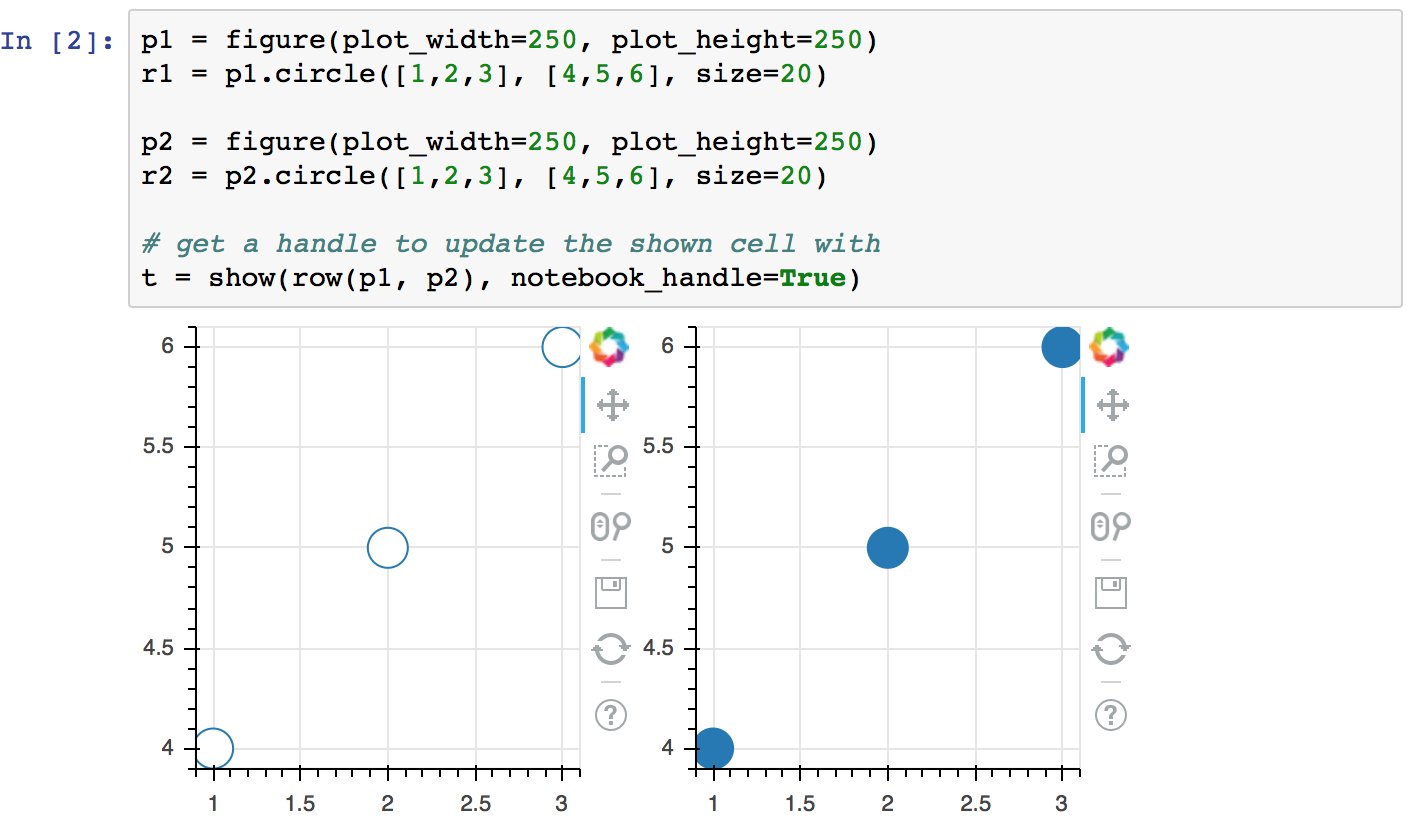More detailed demonstrations of using notebook handles can be found in the following example notebooks:

Jupyter Interactors¶

It is possible to drive updates to Bokeh plots using Jupyter notebook widgets, known as interactors. The key doing this is the push_notebook() function described above. Typically it is called in the update callback for the interactors, to update the plot from widget values. A screenshot of the examples/howto/notebook_comms/Jupyter Interactors.ipynb example notebook is shown below: